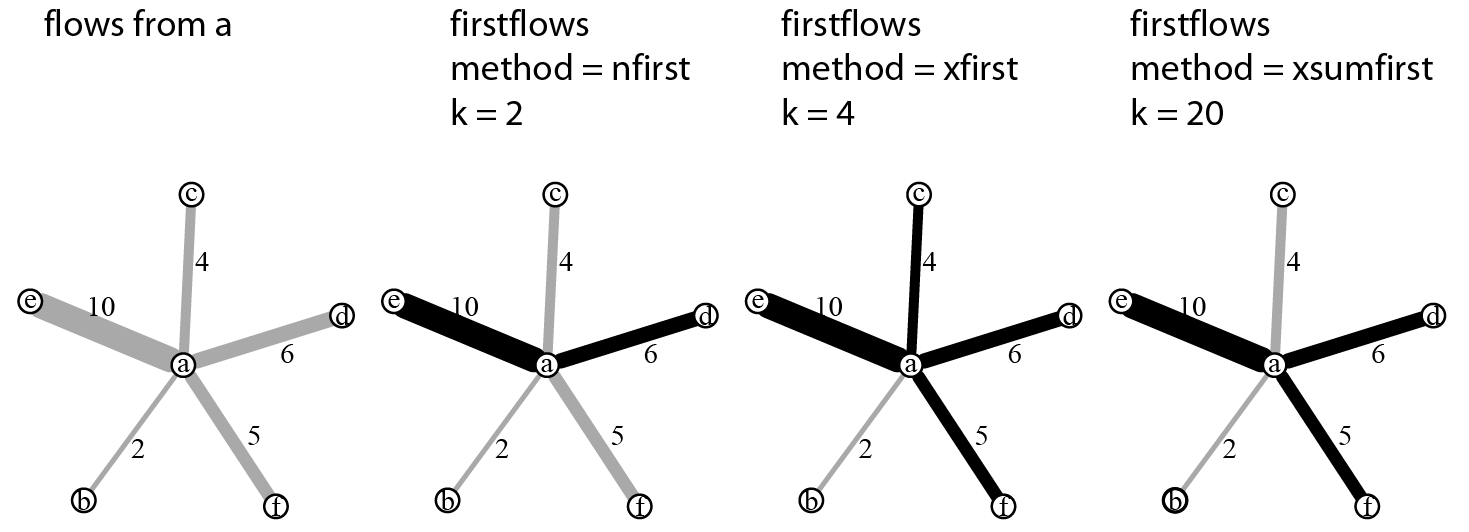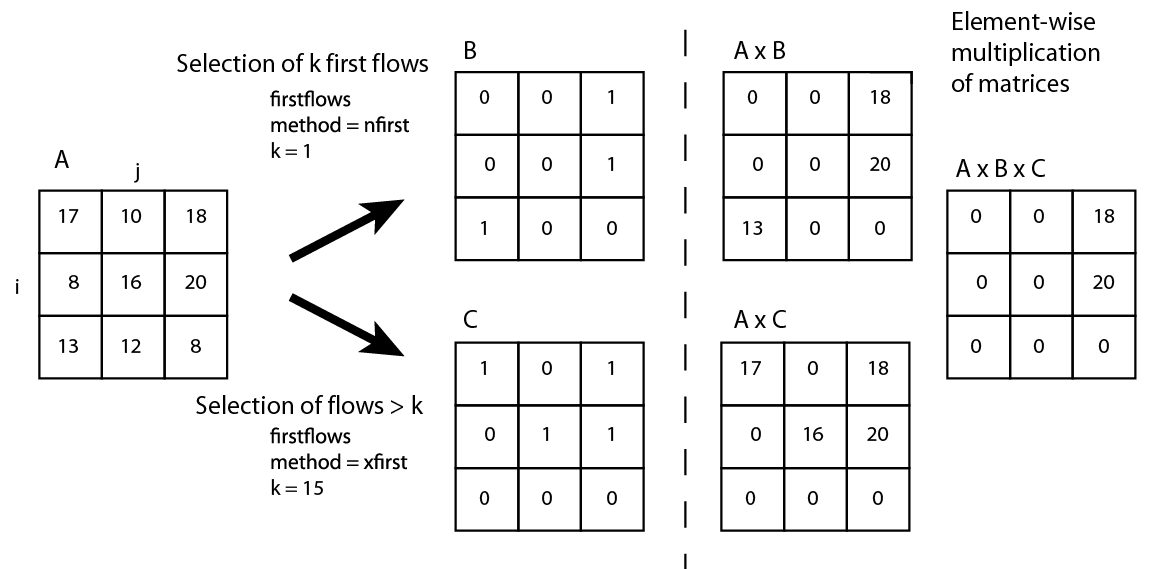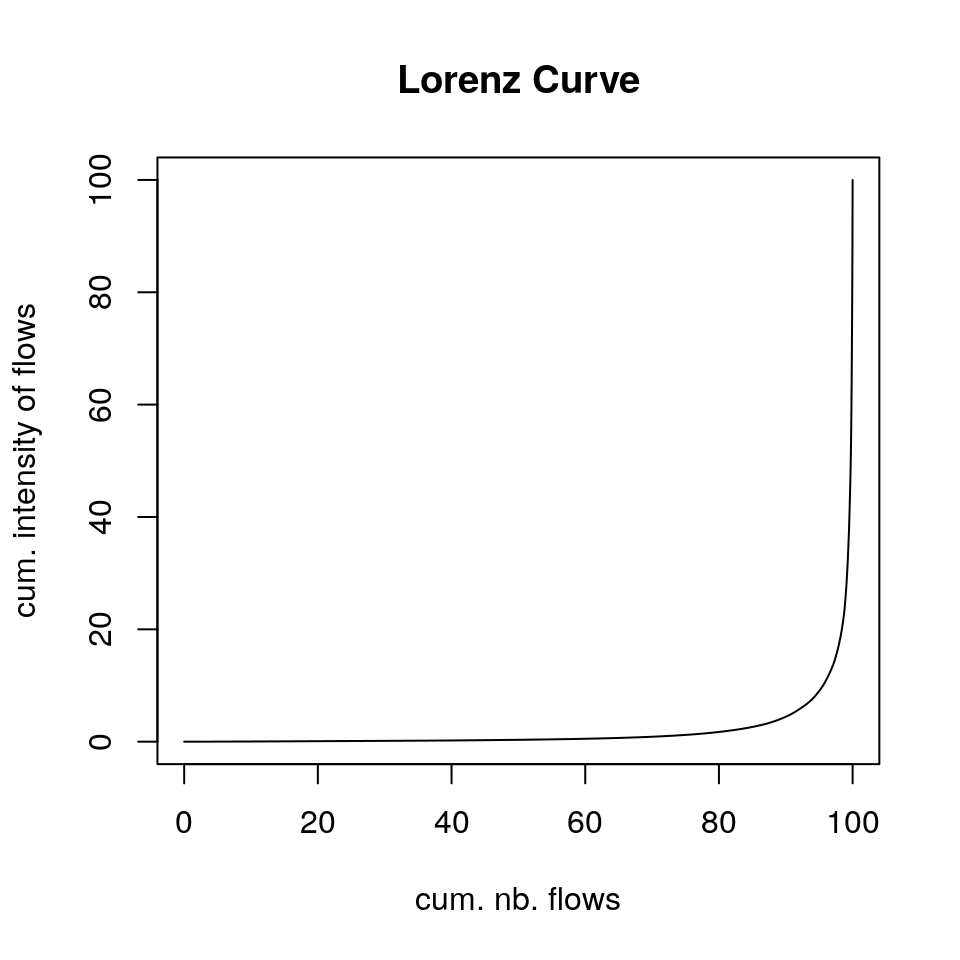# Introduction

The flows package contains functions that select flows, provide statistics on selections and propose map and graph visualisations.
The first part of the vignette reminds several methods of flow selection, the second part presents the main functions of the package and the last one proposes an exemple of analysis based on commuters data in the French Grand Est region.

# Analysis of geographic flows: issues and methods

In the field of spatial analysis, working on flows supposes to focus on the relationships between places rather than on their characteristics. Analysis and flow representation often assume a selection to ease the interpretation.

One of the first method developed was the so-called dominant flows (or nodal regions) proposed by Nystuen and Dacey in 1961 (Dacey (1961)). Working on telephone flows between cities in the Seattle area, they sought to highlight hierarchy between locations. According to this method, a place i is dominated by a place j if two conditions are met:

1. the most important flow from i is emitted towards j;

2. the sum of the flows received by j is greater than the sum of the flows received by i.

This method creates what is called in graph theory a tree (acyclic graph) or a forest (a set of unconnected trees) with three types of nodes: dominant, dominated and intermediate. If the method creates a clear functional hierarchy, its major drawback is to undervalue flows intensities.

Various methods have subsequently been proposed to better reflect this intensity, one of the most frequently used being the so-called major flows: it selects only the most important flows, absolute or relative, either locally or globally. Analysing commuters data between cities, one may choose to select:

• all flows greater than 100;

• the 50 first flows (global criterion);

• the 10 first flows emitted by each city (local criterion).

These criteria can also be expressed in relative form:

• flows that represent more than 10% of the active population of each city (local criterion);

• flows that take into account 80% of all commuters (global criterion).

These methods often highlight hierarchies between places but the loss of information created by the selection is rarely questioned. So it seems useful to propose statistical indicators to assess the volume of lost information and characteristics of the selected flows.

# The package flows

A typical data workflow may be:

1. data preparation;

2. flow selection;

3. statistical data and graphical outputs on the selection made;

4. graph or map representation (dominant flows).

## Data Preparation

Flow data can be found in wide (matrix) or long format (i-j-fij, i.e. origin - destination - flow intensity). As all flows function take flow data in wide format, the `preflows` function transforms a link list into a square matrix. `preflows` has four arguments: a data.frame to transform (`mat`), the origin (`i`), the destination (`j`) and the flow intensity (`fij`).

``````library(flows)
# Import data
data(nav)
``````##     i namei      wi   j                 namej          wj         fij
## 1 001 Paris 5599722 001                 Paris 5599722.265 1698.155329
## 2 001 Paris 5599722 048                Troyes   75561.974    3.909858
## 3 001 Paris 5599722 129                  Sens   24625.065  286.788719
## 4 001 Paris 5599722 529              Vouziers    2119.563    4.047245
## 5 001 Paris 5599722 025                 Dijon  164439.563    5.406881
## 6 001 Paris 5599722 752 Saint-Julien-du-Sault    1048.426    8.097588``````
``````# Prepare data
myflows <- prepflows(mat = nav, i = "i", j = "j", fij = "fij")
myflows[1:4,1:4]``````
``````##          001         009         020         024
## 001 1698.155      0.0000      0.0000      0.0000
## 009    0.000 298895.3551    402.2043    281.4378
## 020    0.000    263.9613 154742.7863   3040.1983
## 024    0.000    258.6355   4500.3492 129716.7266``````

## Flow Selection

Three selection methods based on the flow origins are accessible through the `firstflows` function:

• `nfirst`: the `k` first flows from all origins;

• `xfirst`: all flows greater than a threshold `k`;

• `xsumfirst`: as many flows as necessary for each origin so that their sum is at least equal to `k`.

Figure 1: The three methods of the `firstflows` functionBlack links are the selected ones.

Methods taking into account the total volume of flows are implemented in the `firstflowsg` function. They are identical to the ones described above: selection of the `k` first flows, selection of flows greater than `k` and selection of flows such as the sum is at least equal to `k`.

The `domflows` function selects flows based on a dominance test. This function may be used to select flows obeying the second criterion of Nystuen and Dacey method.

All these functions take as input a square matrix of flows and generate binary matrices of the same size. Selected flows are coded 1, others 0. It is therefore possible to combine criteria of selection through element-wise multiplication of matrices (Figure 2).

Figure 2: Flow selection and criteria combinationThe `statmat` function provides various indicators and graphical outputs on a flow matrix to allow statistically relevant selections. Measures provided are density (number of present flows divided by the number of possible flows); number, size and composition of connected components; sum, quartiles and average intensity of flows. In addition, four graphics can be plotted: degree distribution curve (by default, outdegree), weighted degree distribution curve, Lorenz curve and boxplot on flow intensities.

``````# Import data
data(nav)
myflows <- prepflows(mat = nav, i = "i", j = "j", fij = "fij")

# Get statistics about the matrix
statmat(mat = myflows, output = "none", verbose = TRUE)``````
``````## matrix dimension: 159 X 159
## density: 0.1333493
## nb. of components (weak) 1
## nb. of components (weak, size > 1) 1
## sum of flows: 2306585
## min: 0.8795206
## Q1: 4.008417
## median: 9.544442
## Q3: 54.80416
## max: 298895.4
## mean: 688.5328
## sd: 7765.105``````
``````# Plot Lorenz curve only
statmat(mat = myflows, output = "lorenz", verbose = FALSE)````````````# Graphics only
statmat(mat = myflows, output = "all", verbose = FALSE)``````